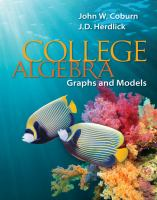Close

# Loose Leaf Version for College Algebra: Graphs & Models

## by Coburn, John, Herdlick, J. D. (John)• ISBN: 9780077430849
• ISBN10: 0077430840

# Loose Leaf Version for College Algebra: Graphs & Models

## by Coburn, John, Herdlick, J. D. (John)

• List Price: \$189.72
• Binding: Ring-bound
• Publisher: McGraw-Hill
• Publish date: 01/28/2011
• ISBN: 9780077430849
• ISBN10: 0077430840
new \$168.91
You save: 11%
FREE economy shipping!
Description: College Algebra: Graphs & Models Chapter R: A Review of Basic Concepts and Skills R.1: Algebraic Expressions and the Properties of Real Numbers R.2: Exponents, Scientific Notation, and a Review of Polynomials R.3: Factoring Polynomials and Solving Polynomial Equations by Factoring R.4: Rational Expressions and Equations R.5: Radicals, Rational Exponents, and Radical Equations Chapter 1: Functions and Graphs 1.1: Rectangular Coordinates, Graphing Circles and Other Relations 1.2: Functions, Function Notation, and the Graph of a Function 1.3: Linear Equations and Rates of Change 1.4: Linear Functions, Special Forms, and More on Rates of Change 1.5: Solving Equations and Inequalities Graphically; Formulas and Problem Solving 1.6: Linear Models and Real Data Chapter 2: Relations, More on Functions 2.1: Analyzing the Graph of a Function 2.2: The Toolbox Functions and Transformations 2.3: Absolute Value Functions, Equations, and Inequalities 2.4: Rational and Radical Functions; More on the Domain 2.5: Piecewise-Defined Functions 2.6: Variation: The Toolbox Functions in Action Chapter 3: Quadratic Functions and Operations on Functions 3.1: Complex Numbers 3.2: Solving Quadratic Equations and Inequalities 3.3: Quadratic Functions and Applications 3.4: Quadratic Models; More on Rates of Change 3.5: The Algebra of Functions 3.6: Composition of Functions and the Difference Quotient Chapter 4: Polynomial and Rational Functions 4.1: Synthetic Division; the Remainder and Factor Theorems 4.2: The Zeros of Polynomial Functions 4.3: Graphing Polynomial Functions 4.4: Graphing Rational Functions 4.5: Additional Insights into Rational Functions Chapter 5: Exponential and Logarithmic Functions 5.1: One-to-One and Inverse Functions 5.2: Exponential Functions 5.3: Logarithms and Logarithmic Functions 5.4: Properties of Logarithms 5.5: Solving Exponential/Logarithmic Equations 5.6: Applications from Business, Finance, and Science 5.7: Exponential, Logarithmic, and Logistic Equation Models Chapter 6: Systems of Equations and Inequalities 6.1: Linear Systems in Two Variables with Applications 6.2: Linear Systems in Three Variables with Applications 6.3: Nonlinear Systems of Equations and Inequalities 6.4: Systems of Inequalities and Linear Programming Chapter 7: Matrices and Matrix Applications 7.1: Solving Linear Systems Using Matrices and Row Operations 7.2: The Algebra of Matrices 7.3: Solving Linear Systems Using Matrix Equations 7.4: Applications of Matrices and Determinants: Cramer's rule, Partial Fractions, and More Chapter 8: Analytic Geometry and the Conic Sections 8.1: A Brief Introduction to Analytic Geometry 8.2: The Circle and the Ellipse 8.3: The Hyperbola 8.4: The Analytic Parabola Chapter 9: Additional Topics in Algebra 9.1: Sequences and Series 9.2: Arithmetic Sequences 9.3: Geometric Sequences 9.4: Mathematical Induction 9.5: Counting Techniques 9.6: Introduction to Probability 9.7: The Binomial Theorem Appendices The Language, Notation, and Numbers of Mathematics Geometry Review with Unit Conversions More on Synthetic Division More on Matrices Deriving the Equation of a Conic Proof Positive - A Selection of Proofs from College Algebra
Expand description